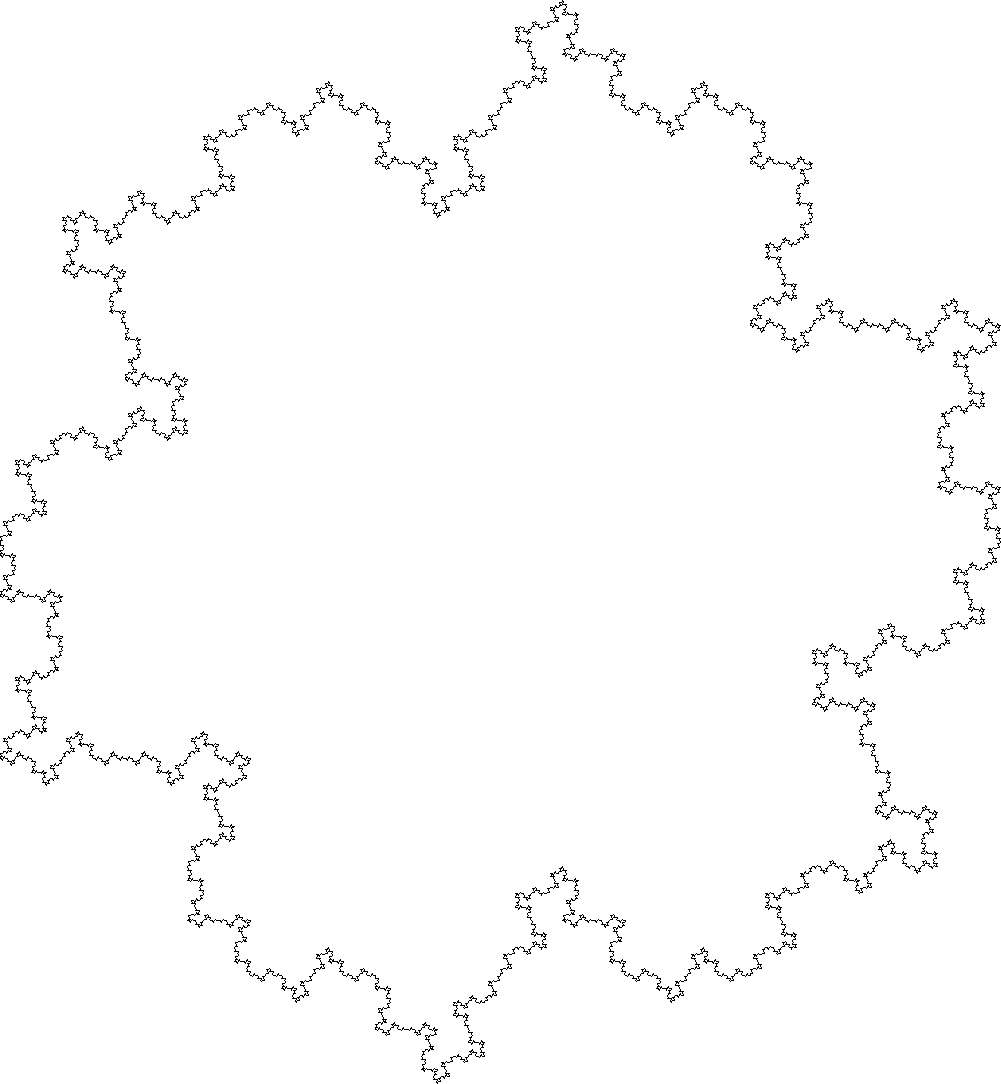# L-system

### Rendered in Python, explained in English

##### By Dany Shaanan
An L-system, or Lindenmayer System, is a rewritting system that can be used to easily create fractals.

So what is being rewritten? A list of instructions. Let's start with "forward". If we'll feed that to a line drawing method, we'll get, obviously, a line:...which is pretty boring, so lets rewrite it; Let's replace "forward" with "forward, 60 degrees left, forward, 120 degrees right, forward, 60 degrees left, forward". If we'll deef that to our line drawing method, we'll get this:For the sake of simplicity, lets use 'f' for forward, and 'r' and 'l' for 60 degrees right and left turns.
Our starting list of instructions is now just 'f', and our replacement rule can be written as 'f' -> 'flfrrflf'.

So if we'll take the last list of instructions, 'flfrrflf', and apply the replacement rule on it, we'll get this: 'flfrrflflflfrrflfrrflfrrflflflfrrflf'. This is hard to read, but it will be drawn into this: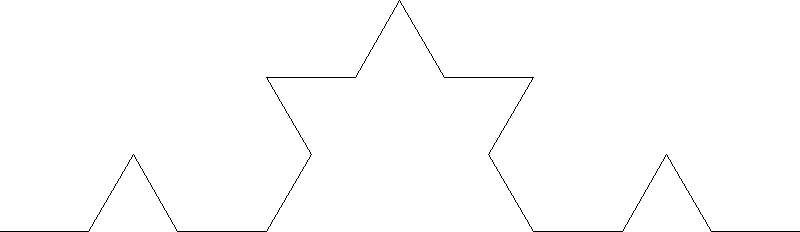Notice that each of the segments in the previous result became similar to the whole previous result. That's the consequance of the replacement rule. By running more iteration of the replacement rule, we'll get a more and more complex line, that includes smaller and smaller instances of the original replacement: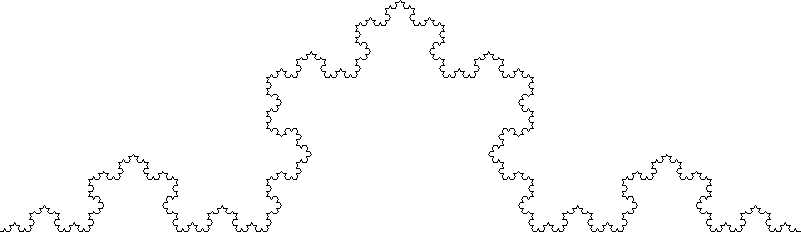Other replacement rules, with different angles and different starting states, can result if various interesting results, as can be seen below.

See the code here

Starting state: "frfrfrf",
Replacement rule: "f" -> "ffrflflfrff",
where 'f' represents 'forward' and 'r' and 'l' represent 90 degrees turns: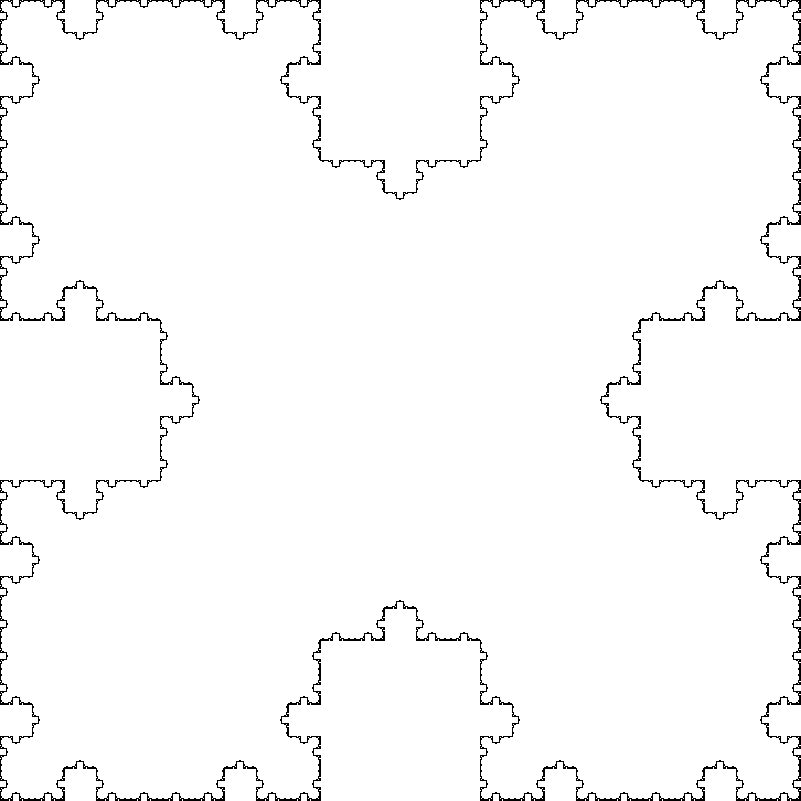Starting state: "fRfRfRf",
Replacement rule: "f" -> "frfllfrf",
where 'f' represents 'forward' and 'r' and 'l' represent 85 degrees turns, and R represent a 90 degree turn: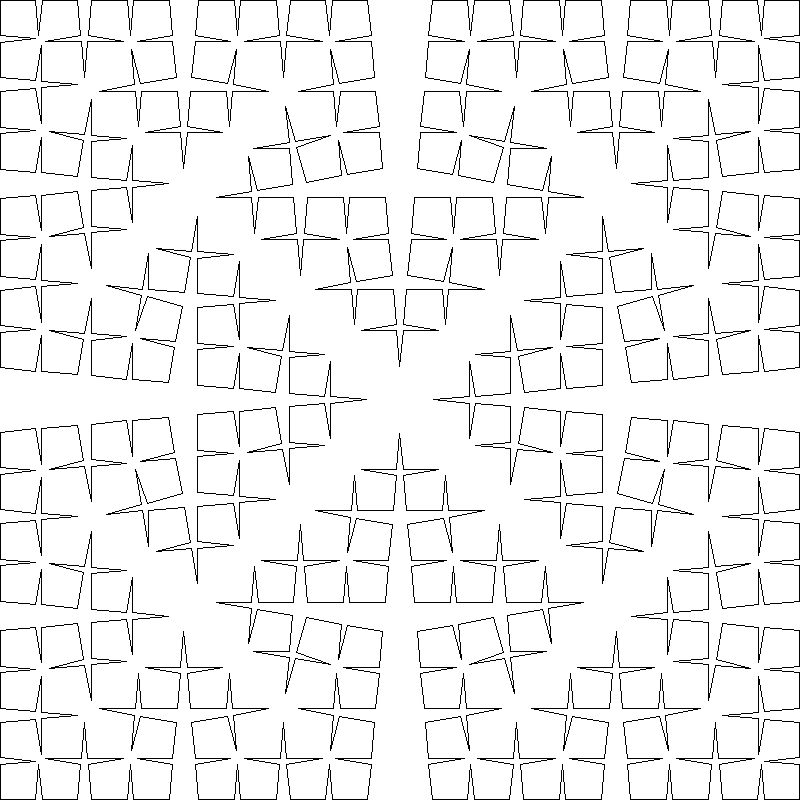Starting state: "flflflf",
Replacement rule: "f" -> "ffrfrfrfrfrflf",
where 'f' represents 'forward' and 'r' and 'l' represent 90 degrees turns: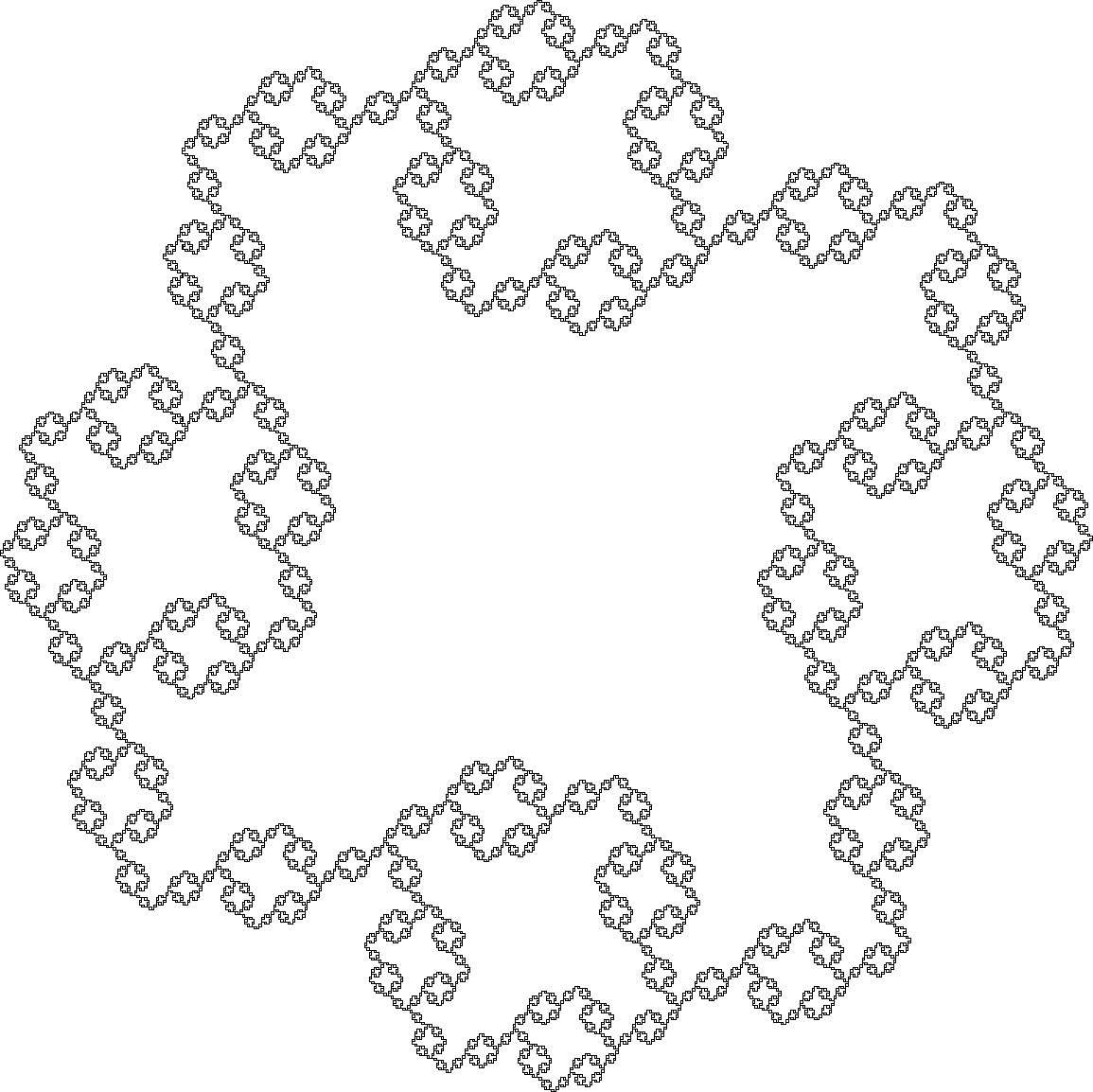Starting state: "a",
Replacement rule: "a" -> "arb", "b" -> "alb",
where 'a' and 'b' represents 'forward' and 'r' and 'l' represent 90 degrees turns: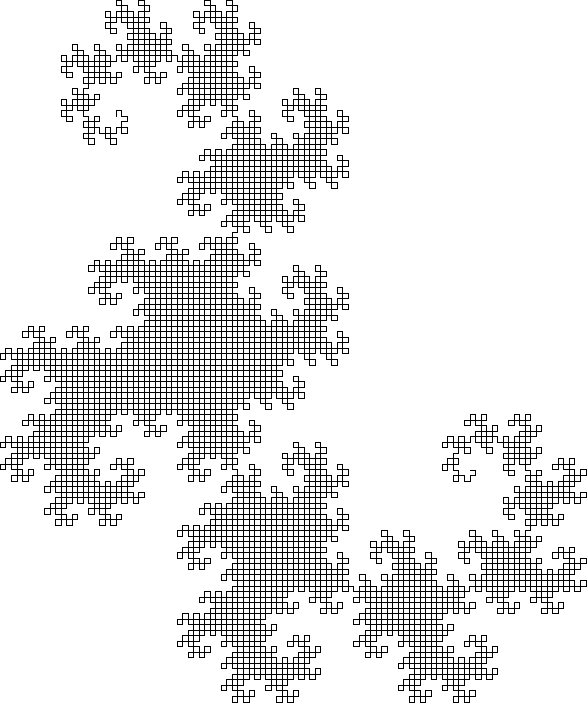Starting state: "frfrfrfrf",
Replacement rule: "f" -> "frfllfrf",
where 'f' represents 'forward' and 'r' and 'l' represent 72 degrees turns: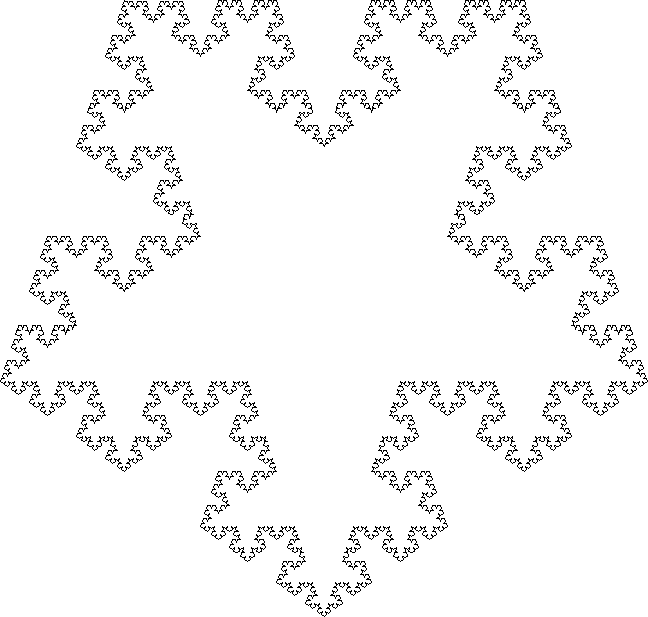Starting state: "rrf",
Replacement rule: "f" -> "rflfflfr",
where 'f' represents 'forward' and 'r' and 'l' represent 90 degrees turns: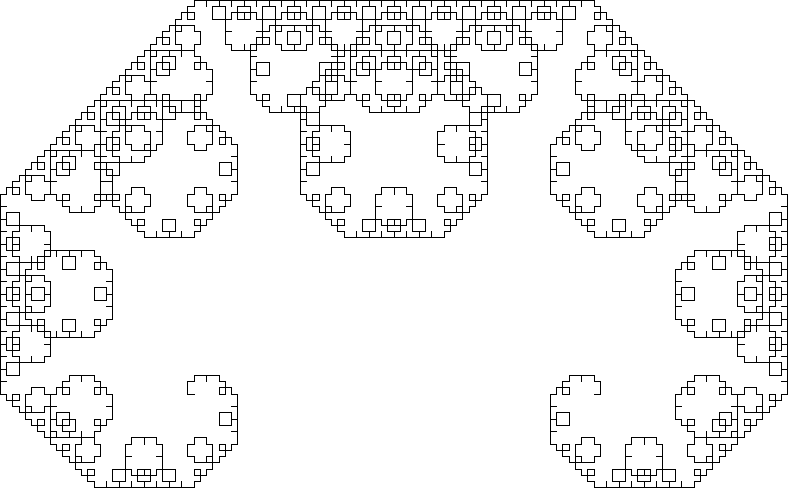Starting state: "f",
Replacement rule: "f" -> "flfrfrflf",
where 'f' represents 'forward' and 'r' and 'l' represent 120 degrees turns: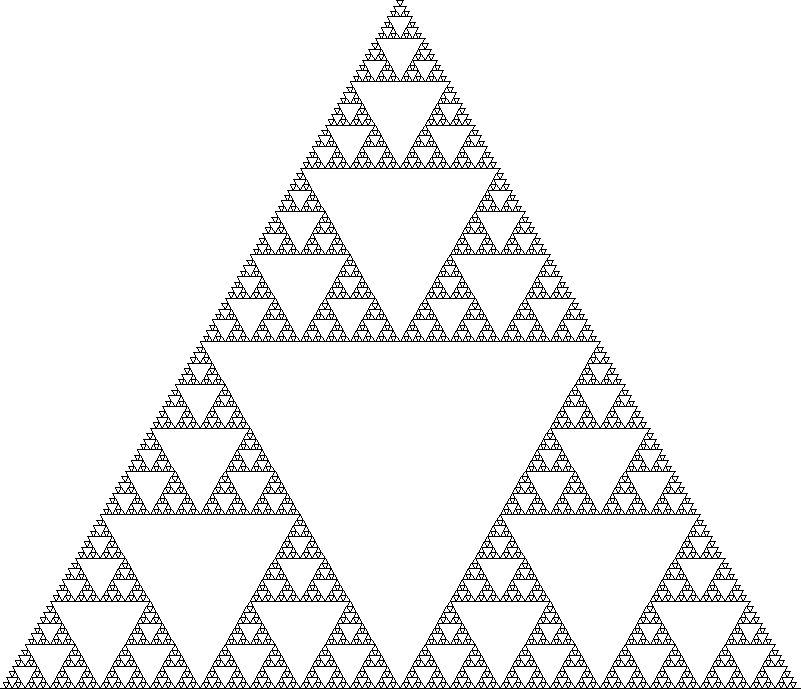Starting state: "a",
Replacement rule: "a" -> "blalb", "b" -> "arbra",
where 'a' and 'b' represents 'forward' and 'r' and 'l' represent 60 degrees turns: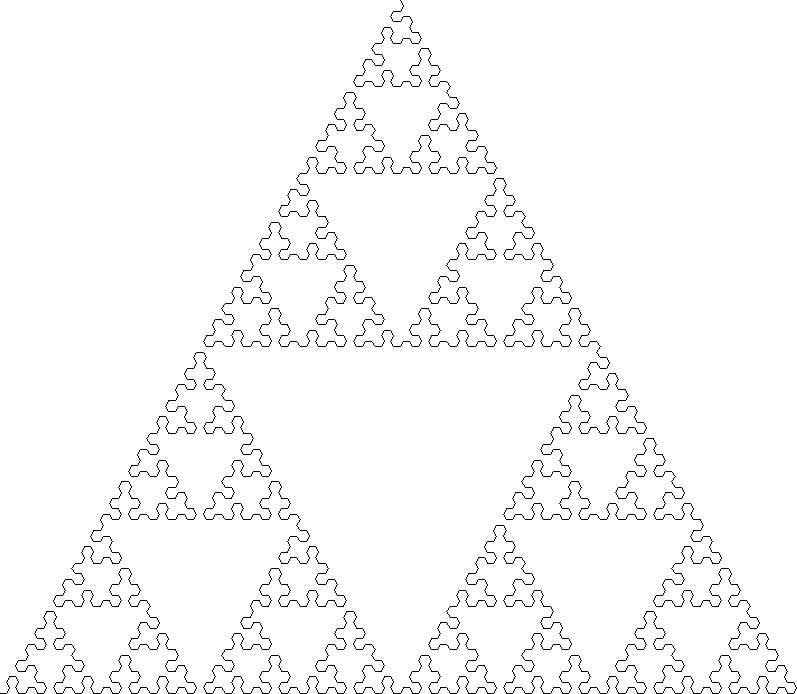Starting state: "arararara",
Replacement rule: "a" -> "aararararallllaa",
where 'a' and 'b' represents 'forward' and 'r' and 'l' represent 90 degrees turns: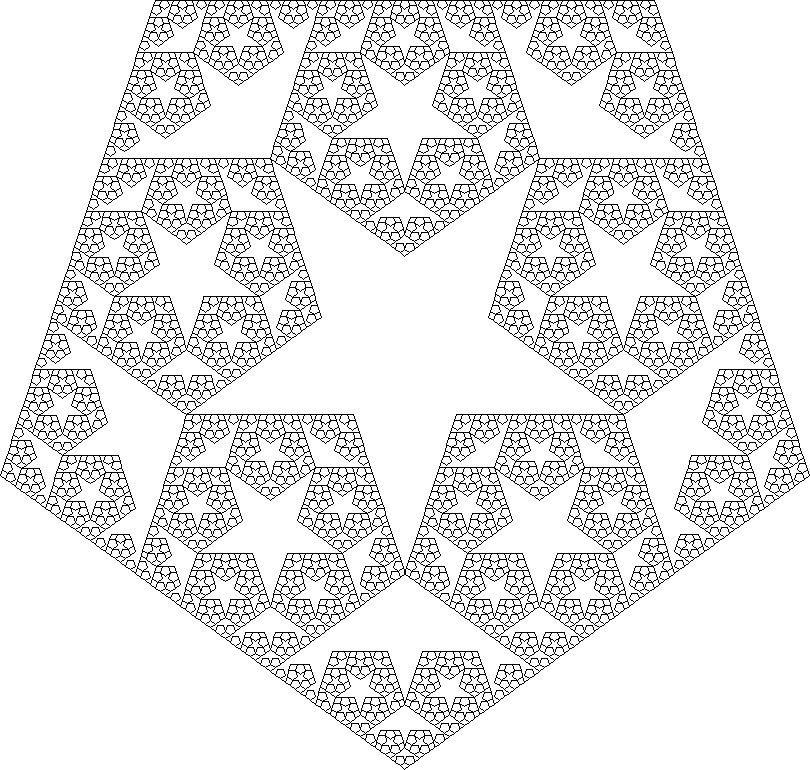Starting state: "frfrfrfrfrf",
Replacement rule: "f" -> "frfllffrrflf",
where 'f' represents 'forward' and 'r' and 'l' represent 60 degrees turns: